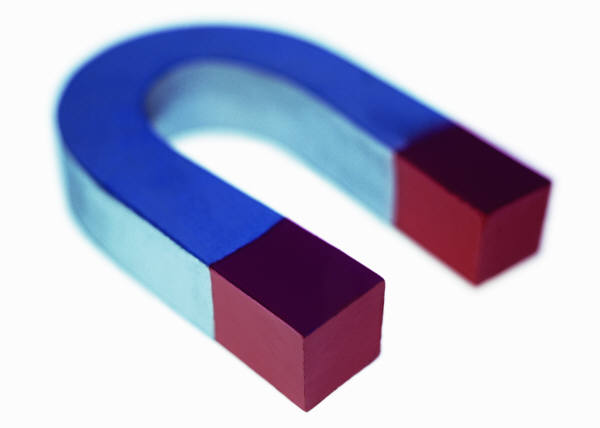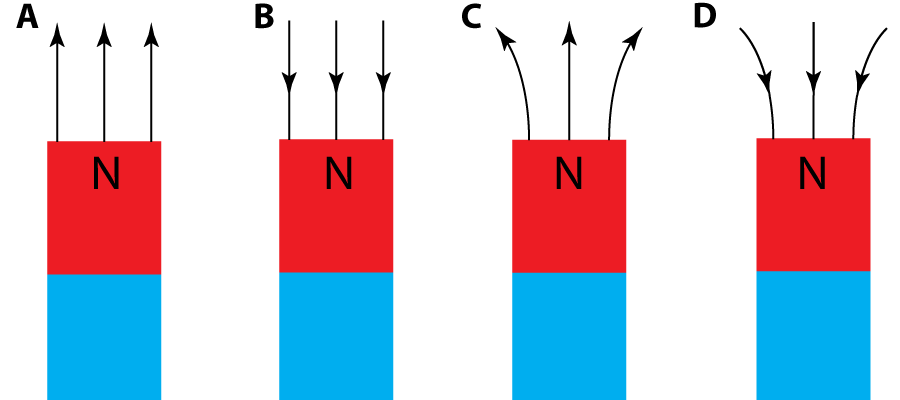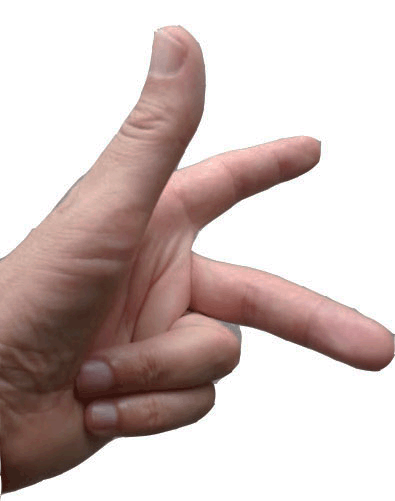OCR GCSE - Magnetism

Page 1

These questions are about magnets:1. Which of these is not a magnetic material?

• A). Aluminium
• B). Steel.
• C). Nickel.
• D). Iron.

2. Which of these diagrams shows the magnetic field lines around the north pole of a magnet?3. When magnets and metal bars are close together they experience forces.

Which row gives the correct description of the effect between these materials?

 North Pole and North Pole North Pole and South Pole North Pole and Iron Bar A). attract repel attract B). repel attract repel C). repel attract attract D). attract attract attract

4. A magnetic compass points North. This shows that...

• A). the Earth is tilted on its axis.
• B). the Earth is spinning.
• C). the Earth has a magnetic field.
• D). the Earth has gravity.

5. A magnetic field line shows the direction of force acting on

• A). a magnetic north pole.
• B). a magnetic south pole.
• C). a bar magnet.
• D). a current carrying wire.

6. Which of these diagrams shows the magnetic field line pattern (red lines) around a single straght wire carrying a current?7. A student cariies out some experiments to see which variables affect the strength of the field near to the wire above. They change one variable at a time, keeping the others contstant. Only 2 of the experiments gave a result.

Which 2 variables were found to affect the field strength?

• A). Distance from the wire, thickness of the wire.
• B). Thickness of the wire, type of metal used.
• C). Type of metal used, current in the wire.
• D). Current in the wire, distance from the wire.

8. What is the name of a long (empty) coil of wire used to produce a magnetic field?

• A). A transformer
• B). A potentiometer
• C). A solenoid
• D). An alternator

9. If an iron bar is placed in the middle of this coil, a strong electromagnet is produced. The magnetic field can then be turned on and off.

This type of magnet is ...

• A). a permanent magnet.
• B). an induced magnet.
• C). a Fleming magnet.
• D). a motor effect magnet.

10. Fleming's Left hand Rule can be used to predict the direction of the force on a wire carrying a current, when placed in a magnetic field.

Which of these correctly identifies the fingers/thumb?

 Force on wire current in wire Magnetic Field direction A thumb first finger second finger B thumb second finger first finger C second finger first finger thumb D first finger thumb second finger